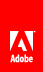# Basics of geometry

Flash Player 9 and later, Adobe AIR 1.0 and later

The flash.geom package contains classes that define geometric objects such as points, rectangles, and transformation matrixes. These classes don’t necessarily provide functionality by themselves; however, they are used to define the properties of objects that are used in other classes.

All the geometry classes are based around the notion that locations on the screen are represented as a two-dimensional plane. The screen is treated like a flat graph with a horizontal (x) axis and a vertical (y) axis. Any location (or point ) on the screen can be represented as a pair of x and y values—the coordinates of that location.

Every display object, including the Stage, has its own coordinate space . The coordinate space is an object’s own graph for plotting the locations of child display objects, drawings, and so on. The origin is at coordinate location 0, 0 (where the x and y-axes meet), and is placed at the upper-left corner of the display object. While this origin location is always true for the Stage, it is not necessarily true for other display objects. Values on the x-axis get bigger going toward the right, and smaller going toward the left. For locations to the left of the origin, the x coordinate is negative. However, contrary to traditional coordinate systems, Flash runtime coordinate values on the y-axis get bigger going down the screen and smaller going up the screen. Values above the origin have a negative y coordinate value). Since the upper-left corner of the Stage is the origin of its coordinate space, most objects on the Stage have an x coordinate greater than 0 and smaller than the Stage width. And the same object has a y coordinate larger than 0 and smaller than the Stage height.

You can use Point class instances to represent individual points in a coordinate space. You can create a Rectangle instance to represent a rectangular region in a coordinate space. For advanced users, you can use a Matrix instance to apply multiple or complex transformations to a display object. Many simple transformations, such as rotation, position, and scale changes, can be applied directly to a display object using that object’s properties. For more information on applying transformations using display object properties, see Manipulating display objects .

#### Important concepts and terms

The following reference list contains important geometry terms:

Cartesian coordinates
Coordinates are commonly written as a pair of number (like 5, 12 or 17, -23). The two numbers are the x coordinate and the y coordinate, respectively.

Coordinate space
The graph of coordinates contained in a display object, on which its child elements are positioned.

Origin
The point in a coordinate space where the x-axis meets the y-axis. This point has the coordinate 0, 0.

Point
A single location in a coordinate space. In the 2-d coordinate system used in ActionScript, the location along the x-axis and the y-axis (the point’s coordinates) define the point.

Registration point
In a display object, the origin (0, 0 coordinate) of its coordinate space.

Scale
The size of an object relative to its original size. When used as a verb, to scale an object means to change its size by stretching or shrinking the object.

Translate
To change a point’s coordinates from one coordinate space to another.

Transformation
An adjustment to a visual characteristic of a graphic, such as rotating the object, altering its scale, skewing or distorting its shape, or altering its color.

X-axis
The horizontal axis in the 2-d coordinate system used in ActionScript.

Y-axis
The vertical axis in the 2-d coordinate system used in ActionScript.// Ethnio survey code removed